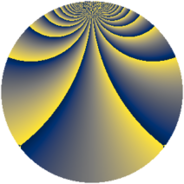# Properties

 Label 2001.2.bhLevel $2001$ Weight $2$ Character orbit 2001.bh Rep. character $\chi_{2001}(157,\cdot)$ Character field $\Q(\zeta_{44})$ Dimension $2400$ Sturm bound $480$

# Related objects

## Defining parameters

 Level: $$N$$ $$=$$ $$2001 = 3 \cdot 23 \cdot 29$$ Weight: $$k$$ $$=$$ $$2$$ Character orbit: $$[\chi]$$ $$=$$ 2001.bh (of order $$44$$ and degree $$20$$) Character conductor: $$\operatorname{cond}(\chi)$$ $$=$$ $$667$$ Character field: $$\Q(\zeta_{44})$$ Sturm bound: $$480$$

## Dimensions

The following table gives the dimensions of various subspaces of $$M_{2}(2001, [\chi])$$.

Total New Old
Modular forms 4880 2400 2480
Cusp forms 4720 2400 2320
Eisenstein series 160 0 160

## Trace form

 $$2400q - 8q^{2} + 8q^{8} + O(q^{10})$$ $$2400q - 8q^{2} + 8q^{8} + 224q^{16} + 8q^{18} + 44q^{19} - 24q^{23} - 384q^{25} + 112q^{26} + 44q^{29} - 8q^{31} + 56q^{32} + 240q^{36} - 72q^{39} - 8q^{41} + 88q^{43} + 308q^{44} - 156q^{46} + 24q^{47} - 176q^{48} + 224q^{49} + 292q^{50} - 76q^{55} + 220q^{56} - 68q^{58} - 16q^{59} + 72q^{70} + 8q^{72} + 4q^{73} - 88q^{74} + 16q^{75} + 120q^{77} + 240q^{81} + 48q^{82} + 84q^{85} + 8q^{87} - 704q^{88} + 264q^{89} - 32q^{94} + 336q^{95} + 264q^{97} - 392q^{98} + O(q^{100})$$

## Decomposition of $$S_{2}^{\mathrm{new}}(2001, [\chi])$$ into newform subspaces

The newforms in this space have not yet been added to the LMFDB.

## Decomposition of $$S_{2}^{\mathrm{old}}(2001, [\chi])$$ into lower level spaces

$$S_{2}^{\mathrm{old}}(2001, [\chi]) \cong$$ $$S_{2}^{\mathrm{new}}(667, [\chi])$$$$^{\oplus 2}$$